C語言中傳值與傳指針的介紹與區別

更新時間：2019年06月23日 09:58:00   作者：守望我要評論

#include<stdio.h>
void swap(int a,int b)
{
int temp = a;
a = b;
b = temp;
printf("swap a = %d,b = %d\n",a,b);
}
int main(void)
{
int a = 10;
int b = 20;
printf("before swap:a = %d,b = %d\n",a,b);
swap(a,b);
printf("after swap:a = %d,b = %d\n",a,b);
return 0;
}

before swap:a = 10,b = 20
internal swap a = 20,b = 10
after  swap:a = 10,b = 20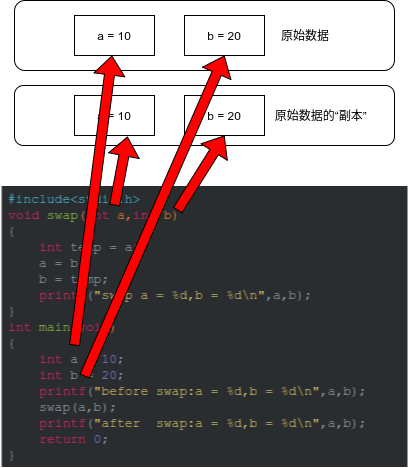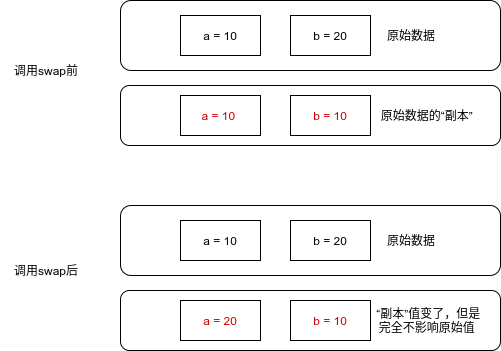#include<stdio.h>
void swap(int *a,int *b)
{
int temp = *a;
*a = *b;
*b = temp;
printf("swap a = %d,b = %d\n",*a,*b);
}
int main(void)
{
int a = 10;
int b = 20;
printf("before swap:a = %d,b = %d\n",a,b);
swap(&a,&b);
printf("after swap:a = %d,b = %d\n",a,b);
return 0;
}

before swap:a = 10,b = 20
swap a = 20,b = 10
after  swap:a = 20,b = 10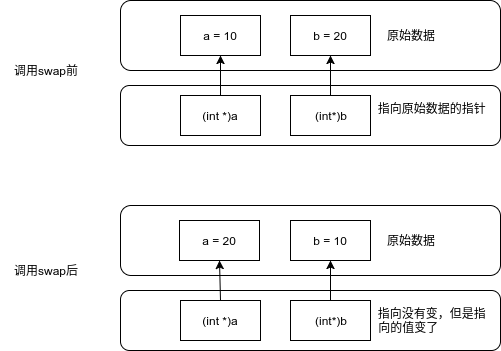#include<stdio.h>
#include<stdlib.h>
void getMemery(int *p)
{
/*申請1024個int大小*/
p = malloc(sizeof(int)*1024);
if(NULL == p)
{
printf("malloc failed\n");
p = NULL;
}
}
int main(void)
{
int *p = NULL;
getMemery(p);
printf("address of p is %p\n",p);
return 0;
}

運行結果：

address of p is (nil)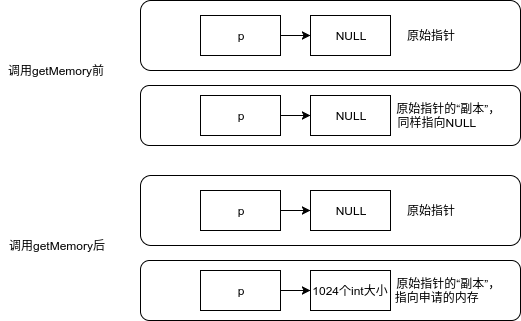#include<stdio.h>
#include<stdlib.h>
void getMemery(int **p)
{
/*申請1024個int大小*/
*p = malloc(sizeof(int)*1024);
if(NULL == *p)
{
printf("malloc failed\n");
*p = NULL;
}
}
int main(void)
{
int *p = NULL;
getMemery(&p);
printf("address of p is %p\n",p);
free(p);
p = NULL;
return 0;
}

address of p is 0x144f010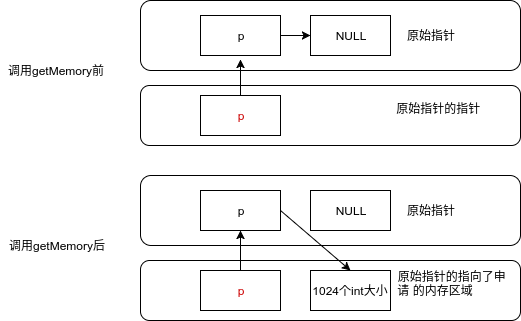• 函數的形參都是原數據的“副本”，因此在函數內無法改變原數據
• 函數中參數都是傳值，傳指針本質上也是傳值
• 如果想要改變入參內容，則需要傳該入參的地址（指針和引用都是類似的作用），通過解引用修改其指向的內容
• 以上結論不限于C語言

• 如何實現不借助第三個變量，交換兩個整數的值？
• 結合本文，理解C++/Java中所謂的傳引用